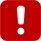# Subnets

In the phone system, we have one large network, spanning a whole country. We could also say that this network is subdivided into smaller, sub-networks -- one for each city, approximately.

So, when we have several phone numbers, how can we tell if they are from the same geographical area, or sub-network? We get this information from the area code . When we have two numbers, such as (323) 337-5578 and (323) 823-8461, we can immediately tell they're both from the same town, or from the same part of the country.

The same goes for IP addresses, but with a slight twist.

Every IP address is composed of two parts -- The network identifier and the host identifier . The network identifier is the left side of the address. For instance, in the address 192.168.0.1 , the first three octets (parts) are the network identifier. So, the addresses for all hosts (computers) on the same network will start with 192.168.0.x .

This can be referred to as a subnet. A subnet is a portion of a network which shares a common network identifier. So, all computers which are (A) on the same network (physically interconnected) and (B) whose addresses start with the same host identifier , belong to the same subnet . Just like (323) 337-5578 and (323) 823-8461 belong to the same area code, so do 192.168.0.1 and 192.168.0.72 belong to the same subnet .

## How Many Hosts Can a Subnet Contain?

With a local telephone number, we can quite easily tell the theoretical limit. If we have 7 unique digits per phone number (excluding the area code, which is shared by all numbers), we can theoretically have up to 9,999,999 phone numbers in the same area code.Of course this isn't accurate -- if we have emergency numbers such as 911, we lose significant portions of this range. You cannot have any number which starts with the digits 911 -- so you lose 9,999 potential numbers, which now cannot be assigned.

With IP addresses, the limitation is slightly different. Each octet in an IP address can range from 0 to 255. This is because an octet is composed of 8 bits. In binary notation, 8 bits can be any combination between 00000000 and 11111111. When you convert these values to decimal notation, 00000000 remains 0 (naturally), and 11111111 equals 255 . Hence, our range -- 0 to 255 per octet.

So, if we selected a network identifier composed of 3 octets -- such as 192.168.0.x -- we have just one octet left for the host identifier . The host identifier must be unique for each host on the network. This means we can have up to 256 hosts per each such subnet.

What if we want to have more than 256 hosts in our subnet? In this case, we must use a network identifier which is composed of less octets. If we use only the first two octets as the network identifier, and use the last two octets as the host identifier, we can have up to 65,536 hosts on the same subnet .It may happen that an octet in a subnet mask will contain a number which is somewhere between 0 and 255 (such as 224). This is relatively rare, and further limits the number of available addresses in the subnet. However, this is beyond the scope of this application note.